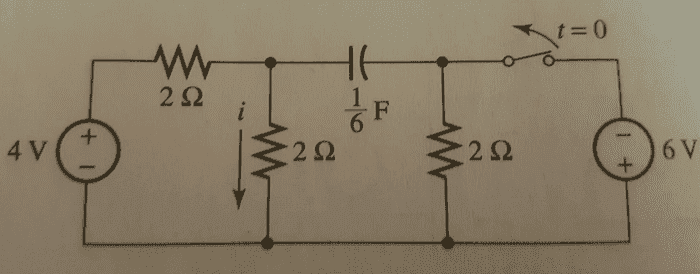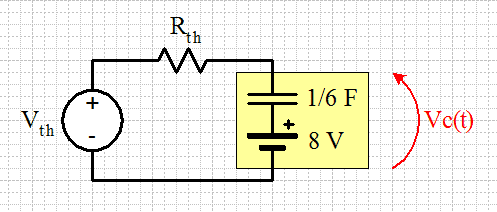# RC circuit, finding voltage across a capacitor

## Homework Statement

I am trying to find the capacitor voltage in the following picture:
Find i(t) in the figure below. Assume dc steady state conditions are valid for t<=0.i=c(dv/dt)
KCL

## The Attempt at a Solution

At t<0 I found Vc to be 8 volts which should be correct according to the book. I am confused at the part after the switch is flipped though. I called the node between the two resistors and the capacitor "v" and used nodal analysis. So (v-4)/2 + v/2 + c(dv/dt)=0. I think my problem is that I am not accounting for the two ohm resistor that is in the branch with the capacitor, but I am not sure how to add that to my equation. My current equation gives me the wrong answer, any help would be appreciated. Oh and I'm trying to get a differential equation to solve, not sure what that method is called.

Last edited:

SammyS
Staff Emeritus
Homework Helper
Gold Member

## Homework Statement

I am trying to find the capacitor voltage in the following picture:i=c(dv/dt)
KCL

## The Attempt at a Solution

At t<0 I found Vc to be 8 volts which should be correct according to the book. I am confused at the part after the switch is flipped though. I called the node between the two resistors and the capacitor "v" and used nodal analysis. So (v-4)/2 + v/2 + c(dv/dt)=0. I think my problem is that I am not accounting for the two ohm resistor that is in the branch with the capacitor, but I am not sure how to add that to my equation. My current equation gives me the wrong answer, any help would be appreciated. Oh and I'm trying to get a differential equation to solve, not sure what that method is called.
Please give the complete statement of the problem. We shouldn't be expected to guess.

Please give the complete statement of the problem. We shouldn't be expected to guess.
I added it. I wanted to find the voltage in the capacitor so i could the find the voltage in the branch parallel to i(t) and then divide by the resistance to get my answer,

SammyS
Staff Emeritus
Homework Helper
Gold Member
I added it. I wanted to find the voltage in the capacitor so i could the find the voltage in the branch parallel to i(t) and then divide by the resistance to get my answer,
That still doesn't look very complete.

What is the situation for t >0 ?

During which time frame is the switch open?

During which time frame is the switch closed?

Doe you need to find the capacitor voltage as function of time, or at some particular time, or after a sufficiently long time ?

That still doesn't look very complete.

What is the situation for t >0 ?

During which time frame is the switch open?

During which time frame is the switch closed?

Doe you need to find the capacitor voltage as function of time, or at some particular time, or after a sufficiently long time ?
For t>0 the switch is opened and stays open forever. The switch is closed at t<=0 and assumed to be closed for a long enough time that the circuit is in dc steady state. Since the question wants i(t) i would want to find capacitor voltage as a function of time.

gneill
Mentor
If you want to solve for the capacitor voltage with respect to time I might suggest that you replace the voltage source and resistor network with its Thevenin equivalent as "seen" by the capacitor. That should make the circuit easier to work with.

Then model the charged capacitor (its initial state at t = 0) with an uncharged capacitor in series with a DC source equal to the capacitor's initial voltage. So you'll get something like this:Of course you're still going to have to find a way to determine your current ##i##, since it isn't represented in this Thevenin version. Have you considered writing mesh equations (KVL loops)?

•timnswede
If you want to solve for the capacitor voltage with respect to time I might suggest that you replace the voltage source and resistor network with its Thevenin equivalent as "seen" by the capacitor. That should make the circuit easier to work with.

Then model the charged capacitor (its initial state at t = 0) with an uncharged capacitor in series with a DC source equal to the capacitor's initial voltage. So you'll get something like this:
View attachment 104981

Of course you're still going to have to find a way to determine your current ##i##, since it isn't represented in this Thevenin version. Have you considered writing mesh equations (KVL loops)?

OK I got Rth and Voc. I calculated Rth to be 3 ohms and Voc (or Vth) to be 2 V. My picture looks the same as yours except I did not add the 8 volts in, and I still used nodal analysis at the node between the capacitor and the Rth. So my nodal equation was (v-2)/3 + C(dv/dt). I solved this first order DE and got v=ce^(-2t) + 2. I then used the initial condition that the capacitor voltage is 8 V when t=0 and got v=6e^(-2t) + 2. From that I got the capacitor current which is i=-2e^(-2t). I multiplied that by the two ohm resistor in the right branch in the original t>0 circuit and added that voltage to the capacitor voltage. So that voltage will be the same as the the middle branch voltage where i(t) is and I got the right answer, e^(-2t)+1.

Sorry if that was a bit confusing to read, but it worked out so I appreciate the tip, but what I am confused about is as to why my original nodal analysis equation (v-4)/2 + v/2 + c(dv/dt)=0 did not work out as shouldn't the current through the capacitor, c(dv/dt), be the same as the current in the right most 2 ohm resistor, so the current in the right branch is just c(dv/dt)?

gneill
Mentor
Sorry if that was a bit confusing to read, but it worked out so I appreciate the tip, but what I am confused about is as to why my original nodal analysis equation (v-4)/2 + v/2 + c(dv/dt)=0 did not work out as shouldn't the current through the capacitor, c(dv/dt), be the same as the current in the right most 2 ohm resistor, so the current in the right branch is just c(dv/dt)?
As you surmised, the problem lies with not accounting for the effect of the resistor in the capacitor branch. The v in your node equation is the potential at the node, not that across the capacitor. That node potential is across the entire branch (cap + resistor).

You could place another node in the branch (between the cap and resistor) and then write another node equation, so two differential equations. The current through the cap would then be ##C\frac{d}{dt}(v1 - v2)##.

•timnswede
As you surmised, the problem lies with not accounting for the effect of the resistor in the capacitor branch. The v in your node equation is the potential at the node, not that across the capacitor. That node potential is across the entire branch (cap + resistor).

You could place another node in the branch (between the cap and resistor) and then write another node equation, so two differential equations. The current through the cap would then be ##C\frac{d}{dt}(v1 - v2)##.
OK thanks! One last question, how would I change my node equation as if I solve it I get a time constant of 1/6, but it should be 1/2? Would i use the impedance of the capacitor?

gneill
Mentor
OK thanks! One last question, how would I change my node equation as if I solve it I get a time constant of 1/6, but it should be 1/2? Would i use the impedance of the capacitor?
I'm not sure that I understand your question. It sounds like you arrived at the correct answer in your post #7. However, in general you don't use the impedance of the capacitor (which is frequency dependent) when solving a transient response differential equation. The exception is when you use the Laplace Domain impedance and use Laplace Transforms to solve the circuit (which is actually a pretty easy yet very powerful method).

I'm not sure that I understand your question. It sounds like you arrived at the correct answer in your post #7. However, in general you don't use the impedance of the capacitor (which is frequency dependent) when solving a transient response differential equation. The exception is when you use the Laplace Domain impedance and use Laplace Transforms to solve the circuit (which is actually a pretty easy yet very powerful method).
I was wondering about what you said about using two nodal equations and having a node between the capacitor and resistor, would my nodal equation for the node between the two resistor and capacitor be right, (v-4)/2 + v/2 + c(dv/dt)=0, as that simplifies to dv/dt + 6v = 12, which when solved would give me a time constant of 1/6, not 1/2 which is the right answer. So I was wondering if I would need to use the laplace domain impedance to solve the two equations.

gneill
Mentor
I was wondering about what you said about using two nodal equations and having a node between the capacitor and resistor, would my nodal equation for the node between the two resistor and capacitor be right, (v-4)/2 + v/2 + c(dv/dt)=0, as that simplifies to dv/dt + 6v = 12, which when solved would give me a time constant of 1/6, not 1/2 which is the right answer. So I was wondering if I would need to use the laplace domain impedance to solve the two equations.

(v-4)/2 + v/2 + c(dv/dt)=0 does not take into account the resistor in series with the capacitor. The "v" in c(dv/dt) mist be the voltage across the capacitor, so this node equation is assuming that the resistor does not exist and that the capacitor is instead connected directly between the node and the reference node (which is not the case).

To use two nodes (one at each end of the capacitor), call them v1 and v2, the voltage across the capacitor would be given by v1 - v2. Thus the current through the capacitor would be C d(v1 - v2)/dt = C (dv1/dt) - C (dv2/dt).